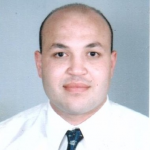Forum Options

Home Forums FIBER dispersion compensationdispersion compensation

Hi!
Using MATLAB compnent dispersion compensation, the result is wrong.
Dispersion compensation expression:H’=exp(j*w^2*beta2*L/2)
use SSMF,：beta2 =-20 L=200km， Not considering attenuation and non-linearity

MATLAB code:
OutputPOrt1 = InputPort1;
for i = 1:length(InputPort1.Sampled.Signal)
OutputPort1.Sampled.Signal(i)=InputPort1.Sampled.Signal(i)*exp(1j*beta2*L/2*InputPort1.Sampled.Frequency(i)*InputPort1.Sampled.Frequency(i));
end

Where is wrong? ? ? Why can not compensate

Responses (7):

• #47375zheng kai
Participant

Hi!
Using MATLAB compnent dispersion compensation, the result is wrong.
Dispersion compensation expression:H’=exp(j*w^2*beta2*L/2)
use SSMF,：beta2 =-20 L=200km， Not considering attenuation and non-linearity

MATLAB code:
OutputPOrt1 = InputPort1;
for i = 1:length(InputPort1.Sampled.Signal)
OutputPort1.Sampled.Signal(i)=InputPort1.Sampled.Signal(i)*exp(1j*beta2*L/2*InputPort1.Sampled.Frequency(i)*InputPort1.Sampled.Frequency(i));
end

Where is wrong? ? ? Why can not compensate

• #47420send both matlab file and .osd file in compressed folder, I might be able to help

thanks
Mohamed Abouseif

• #47427zheng kai
Participant

zip file

Attachments:
• #47439Hi Zheng,
I have checked you project,
I think I got the error.
note that the output of PIN photodetector is time domain signal. so you can not multiply time domain with transfer function in frequency domain!!
So the solution, you have to
convert the output of PIN to frequency domain then multiply by H(omega).
hope that helps,
thanks
Mohamed Abouseif

• #47440zheng kai
Participant

Hi Mohamed Abouseif，
Sampled signal domain is frequency in MATLAB Component.
Can you give me a solution?

• #47441zheng kai
Participant

Hi Mohamed Abouseif，
I try to fft transform PIN output, and then multiplied by H, the result is wrong.

• #47454Zheng,
you need to pay attention about domain of calculation.
after fft pls make sure to compare it with actual frequency domain of the input. in other words, try with something known first (for example Gaussian pulse, or anything similar..) then do the multiplication also make sure again from the results (it is as simple as multiplication)
then return back to time domain by ifft, again make sure from the output is correct. then afterwards, use this matlab component in your project.
be patient in checking each step. keep in your mind it is just a multiplication process!!!! but in frequency domain

• #47466zheng kai
Participant

Hi Mohamed Abouseif，
Do you have any documentation on multiplying the frequency domain?
Let me refer to it，I am very anxious to use this project。
thanks